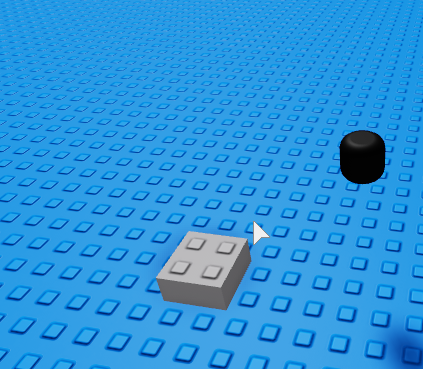# 3D Infinite Perlin Noise Isn't Working

Q1. What do you want to achieve?
A1: I want to create 3D perlin noise in Roblox.

Q2. What is the issue?
A1: When I play the game, nothing happens!

1. What solutions have you tried so far?
I have tried removing while true do
wait() from the script so that it wasn’t infinite however that didn’t work. The tutorial I was watching was only 9 months old so I expected it to be working now.
``````while true do
wait()
mapxsize = 32
mapysize = 32
mapzsize = 32
seed = math.random(0,1000000)
noisescale = 20
amplitude = 20
blocksize = 2

for x = 0,mapxsize do
for z = 0,mapzsize do
for u = 0,mapysize do
xnoise = math.noise(y/noisescale,z/noisescale,x/noisescale,seed)*amplitude
ynoise = math.noise(x/noisescale,z/noisescale,x/noisescale,seed)*amplitude
znoise = math.noise(x/noisescale,y/noisescale,x/noisescale,seed)*amplitude

density = xnoise + ynoise + znoise
if density < 10 then
part = Instance.new("Part",workspace.TerrainFolder)
part.Anchored = true
part.Size = Vector3.new(blocksize,blocksize,blocksize)
part.CFrame = CFrame.new(x*blocksize,y*blocksize,z*blocksize)
end
end
end
end
end
``````

Changed the y value to 32, now this happens…You are missing your Y variable I think, on line 13 you wrote “for u = 0,mapysize do” instead of “for y = 0,mapysize do” and in the rest of the script you are using the variable y instead of u, try changing that? Check the output and tell me if there are any errors, if not try checking under the baseplate to see if anything loads under it.

1 Like